# How to Read a Ruler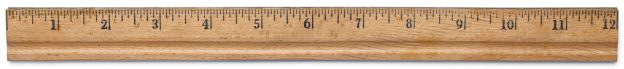## What is a Ruler

A ruler is a device with measurement markings on it used for measuring drawing straight lines. Students, engineers, contractors, and makers use rulers for math, construction, architecture, sewing, landscaping, and more.

According to Dictionary.com, a ruler is a strip of wood, metal, or other material having a straight edge and usually marked off in inches or centimeters, used for drawing lines, measuring, etc.

Several types of rulers include wooden or metal rulers, yardsticks, seamstress tapes, tape measures, carpenters rules, and architects scales.

Rulers have measurements in imperial and metric, imperial-only, or metric-only. Get more information on rulers, including different types and uses, or download and print one of our free printable rulers.

## How to Use a Ruler – Standard Imperial MeasurementsThe markings on a standard ruler represent the fractions of an inch. The markings on a ruler from the start to the 1″ mark are: 116“, 18“, 316“, 14“, 516“, 38“, 716“, 12“, 916“, 58“, 1116“, 34“, 1316“, 78“, 1516“, and 1”. If the measurement is over 1″, simply use the number on the ruler and add the fraction. For instance, if you’re two ticks past the number 3 tick, then the measurement is 3 18“.

### What Do the Markings on a Ruler Mean

Reading a ruler starts with understanding what all the ticks mean. The largest ticks on a ruler represent a full inch, and the distance between each large tick is 1″.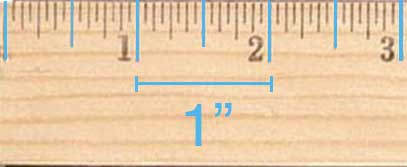The large ticks in between the inch markings are half-inch markings, and the distance between an inch tick and a half-inch tick is 12“.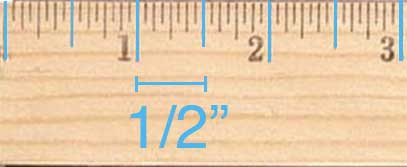The mid-sized ticks between the inch ticks and half-inch ticks are the quarter-inch ticks. The distance between a quarter-inch tick and an inch tick or a half-inch tick is 14“.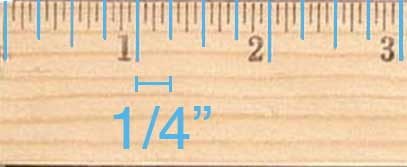The smaller ticks are the eighth-inch ticks and may be the smallest or second smallest markings on the ruler. The distance between an eighth-inch tick and the other larger ticks is 18“.The smallest ticks on a ruler are the sixteenth-inch ticks. The distance between a sixteenth-inch tick and the other larger ticks is 116“.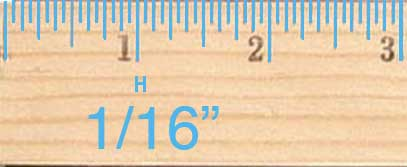## How to Use a Metric Ruler – Metric Measurements

Metric rulers have centimeter and millimeter markings. The larger markings these represent a centimeter.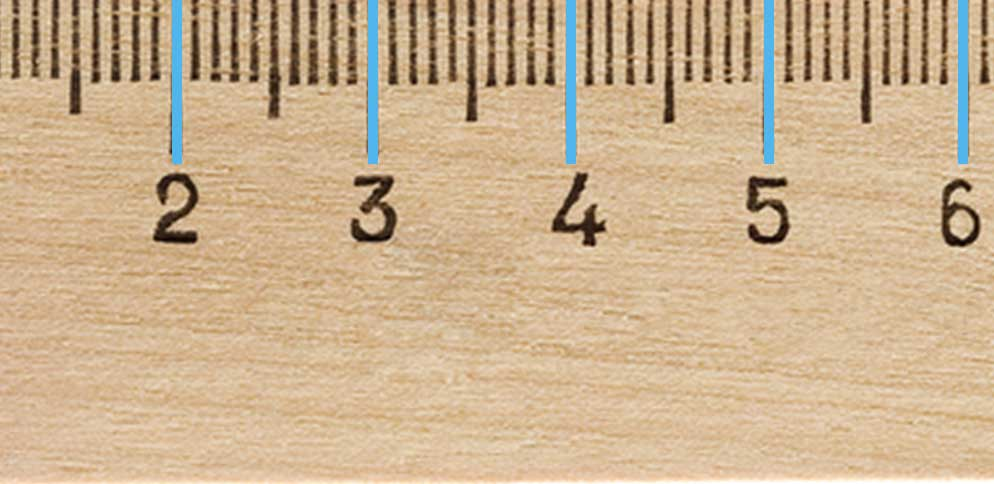The smaller ticks on a metric ruler represent a millimeter. There are 10 millimeters in a centimeter, so there are 9 millimeter ticks between each centimeter tick.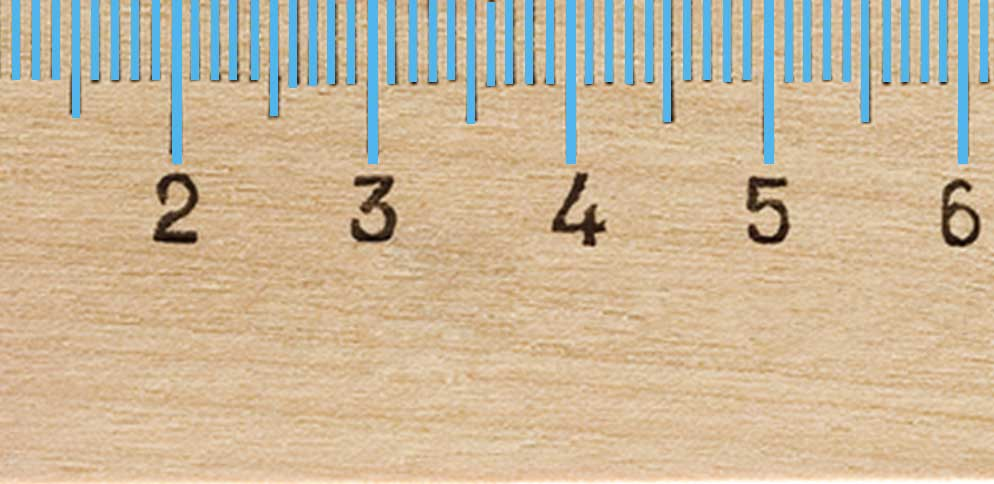## Ruler Measurements: Inch Fractions on a Ruler

These are the measurements and fractions that are on a ruler and the decimal and millimeter metric equivalents. If you need to convert larger inch fractions to decimal or metric, use our inch fraction calculator.### Fraction, Decimal, and Millimeter Equivalent Measurements

Ruler fraction measurements converted to equivalent decimal and millimeter metric values.
Fraction Decimal Millimeters
116 0.0625 1.5875
18 0.125 3.175
316 0.1875 4.7625
14 0.25 6.35
516 0.3125 7.9375
38 0.375 9.525
716 0.4375 11.1125
12 0.5 12.7
916 0.5625 14.2875
58 0.625 15.875
1116 0.6875 17.4625
34 0.75 19.05
1316 0.8125 20.6375
78 0.875 22.225
1516 0.9375 23.8125
1″ 1 25.4

## References

1. Dictionary.com, Ruler, https://www.dictionary.com/browse/ruler